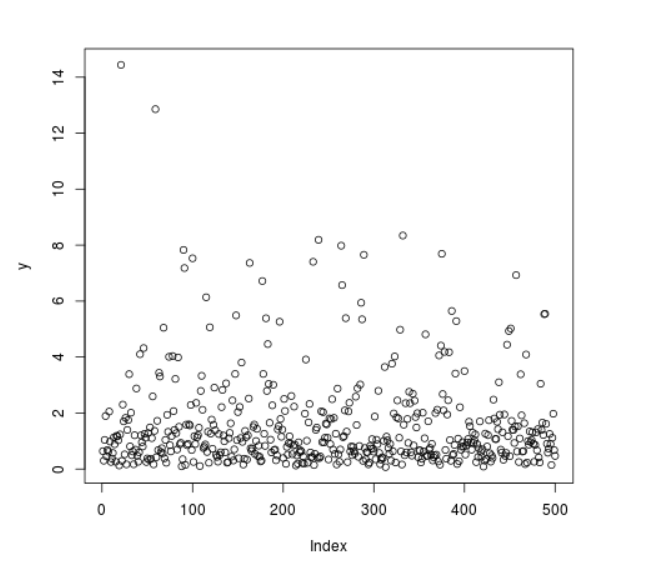# Compute Randomly Drawn Log Normal Density in R Programming – rlnorm() Function

• Last Updated : 25 Jun, 2020

`rlnorm()` function in R Language is used to compute random log normal density among a range of inputs.

Syntax: rlnorm(N)

Parameters:
N: Sample Size

Example 1:

 `# R program to generate ``# random log normal density points`` ` `# Setting seed for``# random number generation``set``.seed(``1000``)`` ` `# Set sample size``N <``-` `20`` ` `# Calling rlnorm() Function``y <``-` `rlnorm(N)``y `

Output:

```  0.6403257 0.2994354 1.0419837 1.8953214 0.4554113 0.6801178 0.6213456
 2.0539211 0.9816646 0.2533159 0.3744010 0.5743659 1.1290552 0.8861471
 0.2628844 1.1853730 1.1677499 1.0252453 0.1291752 1.2375754
```

Example 2:

 `# R program to generate ``# random log normal density points`` ` `# Setting seed for``# random number generation``set``.seed(``1000``)`` ` `# Set sample size``N <``-` `500`` ` `# Calling rlnorm() Function``y <``-` `rlnorm(N)`` ` `# Plot a graph``plot(y)`

Output:My Personal Notes arrow_drop_up